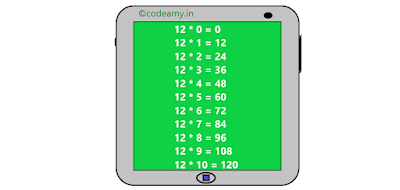# In this C example, you learn about the multiplication table in C program.Multiplication table in c program using loop

When the user enters a number then the number is multiplied with i(iterator) and its value increases from 0 to 10 and prints each.

``````#include<stdio.h>

int main(){
int i,n;
printf("Enter Number to Print Multiplication Table: \n");
scanf("%d",&n);
for(i=0;i<=10;i++)
{
printf("%d * %d = %d \n",n,i,n*i);
}
return 0;
}
``````

Program Output:
```Enter Number to print Multiplication Table:
5
5 * 0 = 0
5 * 1 = 5
5 * 2 = 10
5 * 3 = 15
5 * 4 = 20
5 * 5 = 25
5 * 6 = 30
5 * 7 = 35
5 * 8 = 40
5 * 9 = 45
5 * 10 = 50

--------------------------------```

In this example, I share the code of the multiplication table in C program. Don't forget to share your experience in the comment box.

Happy Coding 😊

1.